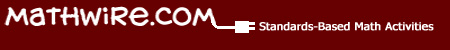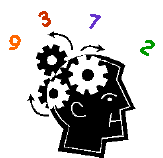Problem Solving: Logic Number Puzzles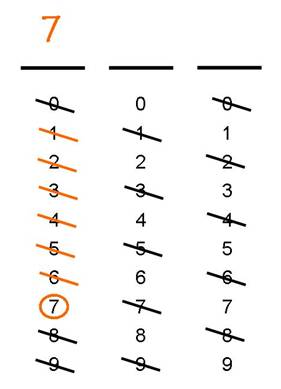## Logic Number Puzzles

These logic number puzzles help students develop strong number sense as they work, clue by clue, to identify the digits of the missing number. The mixed-skills clues incorporate even-odd, less than-greater than, operations (sum, difference), multiples of 5 and 10, geometric terms (octagaon, pentagon, hexagon, quadrilateral, trapezoid, parallelogram), money (quarters, nickels) and measurement (cup, pint, quart, gallon). Students must squeeze every bit of knowledge from each clue to eliminate possible digits until they finally identify the missing digits.

Strategy: Students may solve these problems in many ways, but this organized approach is most effective and easily replicated by students in the future. Students should also feel free to consult math texts, math word walls, etc. for help with measurement clues, geometric terms, etc., as needed.

Students use an organized approach to solving these place value logic number puzzles. Students begin with 0-9 as possible digits for each place value. Each clue allows them to eliminate some of those possibilities. The process continues until there is only one possible digit for each place value.

As a final step, students check their work. As each clue is read, students give a thumbs up if the clue fits their number or a thumbs down if it doesn't. This last step provides immediate feedback to students on the correctness of their reasoning.

Teaching Highlights: The lesson plan format also encourages discussion, moving from whole-class solution to small group guided practice to independent problem solving. Teachers may elect to work closely with struggling students through the guided practice, pulling them to a back table, asking questions and modeling the elimination of digits.

Most students, however, should be encouraged to work in the small group, then independent practice mode. Teachers should resist the urge to lead the whole class through all problems. Students MUST struggle through clues in order to build their own number sense. Instead, schedule time for student-led reviews of particular problems where the student thinks aloud at the overhead and describes how he/she gleaned information from each clue and decided which numbers should be eliminated. Students learn a lot from their peers.

Materials:

• Copy ofLogic Number Puzzle -1 packet for teacher reference which demonstrates the clue by clue process of eliminating possible digits. Use this problem as the whole class presentation. Ask questions to solicit student reasoning after each clue. Summarize student statements as the recorder crosses out eliminated numbers.
•Logic Number Puzzles Template for each student or small group.   Place each chart in a clear sleeve protector so that students can use dry erase markers to cross out numbers after hearing each clue. This template has both 3-digit and 4-digit templates.
• Dry-erase markers and erasers for each group
•Logic Number Puzzle Template transparency
• Copies ofLogic Number Puzzles - 2. Answer Key is included with student handout.
• Copies ofLogic Number Puzzles - 3. Answer Key is included with student handout.
• Copies ofLogic Number Puzzle Template - 2 which has 4 copies of the 3-digit template on one handout.

Introducing the Lesson:

• Distribute copies of theLogic Number Puzzle Template to students and have them place the template in sheet protectors. Distribute dry erase markers and erasers to students.
• Place the Logic Number Puzzle Template transparency in a sheet protector and place on the overhead. Pick a student to act as class recorder at the overhead. Give the student a dry erase marker and eraser to use on the overhead transparency.
• Discuss the Logic Number Puzzle Template with students and talk about how they will use the template to solve the problem, eliminating numbers clue by clue.
• Read the first clue and ask students what the clue tells them about the number. Ask students if there are any digits they may eliminate because of this clue. Once students reach agreement, ask the student at the overhead to eliminate any possibilities as students do the same on their own templates.
• Continue this process, clue by clue, leading the class discussion and helping students identify which digits are eliminated by each clue.
• When all clues have been read and processed, students should have the full number written on the lines above the digits table.• Check work: Tell students that the last process is to check their work. Ask students to look at their number as you reread each clue. They should signal thumbs up, if the number fits the clue; thumbs down if it doesn't. A thumbs down means that students need to rework that clue. All thumbs up means that the students most likely have the correct answer.

Small Group Practice:

• Ask students to erase their Logic Number Puzzle templates.
• Distribute theLogic Number Puzzles - 2 to students.
• Ask groups to work through the first problem, reading the clue aloud, discussing what digits may be eliminated, reaching agreement and eliminating those digits on their templates.
• Circulate around the room as students work to be sure that all students are actively involved and that groups are discussing each clue and reaching agreement on which digits may be eliminated.
• Look for students checking their work, using the thumbs up approach, before signaling that they have completed the problem.
• As each group supplies the correct answer, ask them to erase their templates and begin working on the next problem.
• NOTE: If there are two teachers or a teacher's aide in the classroom, they may pull struggling students into a small group and work with them through the first couple of problems.

Individual Practice:

• When a group finishes all problems, they should be given copies ofLogic Number Puzzles - 3 and theLogic Number Puzzle Template - 2 to record their work, if desired. Using this template allows teachers to collect and review student work.
• Each student should work independently at his/her own pace to solve these problems and record each answer on the handout.
• Circulate around the room as students work to be certain that all students are actively involved and working independently to solve each problem. Intervene, as necessary, to provide help or encouragement.
• Encourage students who finish early to create their own logic number puzzles for classmates to solve.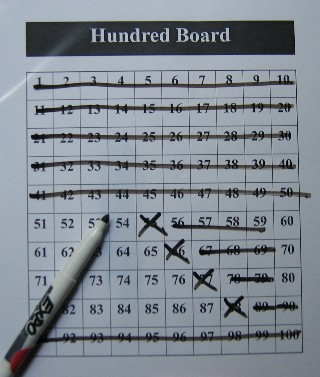## Additional Logic Number Problems

### Hundred Board Logic Problems

Strategy: Students use a hundred board to eliminate numbers after reading each clue. Students must apply their knowledge of even-odd, multiples and place value to successfully eliminate numbers until the solution is revealed.

• Read more about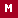Hundred Board Logic Problems and download the teacher packet and instructions.

### Guess the Number

Give students more practice in using a hundred board to solve number clue problems. Once again, students solve the problem clue by clue, eliminating numbers as they go. With this set, however, students must use multiplication, money, and measurement facts to correctly solve the problem and guess the number.

• Download theGuess the Number teacher resource packet which includes 10 2-digit number puzzles plus a template for students to write their own Guess the Number problems.

Sample Problem:Guess the Number-1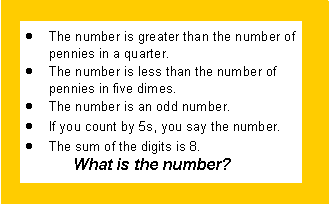Suggested Uses:

• Do Now! or warm-up activity
• Math Center Activity
• Problem solving activity on game days
• Writing original Guess The Number problems for a class collection